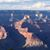Sindhuja Parimi
3

Resistance of a circuit connected in different ways. They are series , parallel,series-parallel and parallel- series.

Now let us see a circuit with series resistanceThe equivalent resistance can be calculated by,

Requivalnt=R1+R2+R3

For n-series connected circuit,the equivalent resistance can be given by,

Requivalnt=R1+R2+R3+..........+Rn

Now let us consider a parallel circuitThe equivalent resistance can be given by,

\frac{1}{R_{equivalent}}=\frac{1}{R_1}+\frac{1}{R_2}+\frac{1}{R_3}

For n-parallel connected circuit,the equivalent resistance can be given by,

\frac{1}{R_{equivalent}}=\frac{1}{R_1}+\frac{1}{R_2}+\frac{1}{R_3}+...............+\frac{1}{R_n}

The series-parallel circuit is given byHere R2 and R3 are connected parallel.These two resistances are connected in series with R1. So the equivalent resistance can be calculated by,

R_{equivalent}=R_1+\left(\frac{R_2R_3}{R_2+R_3}\right)

The parallel-series circuit can be given byHere R1 and R3 are connected in parallel.These two resistors are connected series with R2. So the equivalent resistance can be given by

R_{equivalent}=\left(\frac{R_1R_3}{R_1+R_3}\right)+R_2Vivekanand Vellanki
1
In the parallel case, shouldn't the equivalent resistance between \frac{1}{R} = \frac{1}{R_1} + \frac{1}{R_2} + \frac{1}{R_3}Mahesh Godavarti
1

Resistors can either be connected in series or in parallel.

Everything follows from understanding how two resistors can be connected with each other to create an equivalent new resistor.

Two resistors can either be connected in seriesThis is equivalent to a resistor R with R = R_1 + R_2 .

Or in parallelThis is equivalent to a resistor R with \frac{1}{R} = \frac{1}{R_1} + \frac{1}{R_2} \implies R = \frac{R_1 R_2}{R_1 + R_2} .

Equipped with this, we can tackle any number of resistors connected in any combination.

Let's take three resistors, R_1, R_2, \text{ and } R_3 .

We can select two of them, connect them in either series or parallel to get an equivalent resistor R and connect that in either series or parallel with the third one.

Here are the various combinations.

First select R_1 \text{ and } R_2 and then R_3 .

1. Connect R_1 and R_2 in series. Then connect the equivalent R = R_1 + R_2 with R_3 in series to get a final equivalent resistor of R_1 + R_2 + R_3
2. Connect R_1 and R_2 in parallel. Then connect the equivalent R = \frac{R_1 R_2}{R_1 + R_2} with R_3 in series to get a final equivalent resistor of \frac{R_1 R_2}{R_1 + R_2} + R_3
3. Connect R_1 and R_2 in parallel. Then connect the equivalent R = \frac{R_1 R_2}{R_1 + R_2} with R_3 in parallel to get a final equivalent resistor of \frac{R_1R_2R_3}{R_1R_2+R_2R_3+R_1R_3}

First select R_1\text{ and }R_3 and then R_2.

1. Connect R_1 and R_3 in series. Then connect the equivalent R=R_1+R_3 with R_2 in series to get a final equivalent resistor of R_1+R_3+R_2\ =\ R_1+R_2+R_3
2. Connect R_1 and R_3 in parallel. Then connect the equivalent R=\frac{R_1R_3}{R_1+R_3} with R_2 in series to get a final equivalent resistor of \frac{R_1R_3}{R_1+R_3}+R_2
3. Connect R_1 and R_3 in parallel. Then connect the equivalent R=\frac{R_1R_3}{R_1+R_3} with R_2 in parallel to get a final equivalent resistor of \frac{R_1R_2R_3}{R_1R_2+R_2R_3+R_1R_3}

First select R_2\text{ and }R_3 and then R_1.

1. Connect R_2 and R_3 in series. Then connect the equivalent R=R_2+R_3 with R_1 in series to get a final equivalent resistor of R_1 + R_2 + R_3
2. Connect R_2 and R_3 in parallel. Then connect the equivalent R=\frac{R_2R_3}{R_2+R_3} with R_1 in series to get a final equivalent resistor of \frac{R_2R_3}{R_2+R_3}+R_1
3. Connect R_2 and R_3 in parallel. Then connect the equivalent R=\frac{R_2R_3}{R_2+R_3} with R_1 in parallel to get a final equivalent resistor of \frac{R_1R_2R_3}{R_1R_2+R_2R_3+R_1R_3}

Note that options 1 and 3 in each of the combinations result in the same final equivalent resistance. Therefore, there are five possible equivalent resistors possible using three resistors and connecting them in various series and parallel combinations.

Therefore, the five resistors are:

Options 1, 2 and 3 from the first combination.

Option 2 from the second combination

Option 2 from the third combinationSunita Raman
0
That is a very lucid explanation. Much appreciated. It was the three combinations that was throwing me.